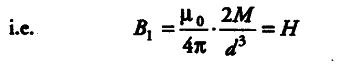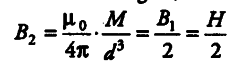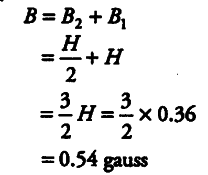# A short bar magnet placed in a horizontal plane

A short bar magnet placed in a horizontal plane has its axis aligned along the magnetic North-South direction. Null points are found on the axis of the magnet at 14 cm from the centre of the magnet. The earth’s magnetic field at the place is 0.36 gauss and the angle of dip is zero.
What is the total magnetic field on the normal bisector of the magnet at the same distance as the null point (i.e. 14 cm) from the centre of the magnet? (At null points, field due to a magnet is equal and opposite to the horizontal component of the earth’s magnetic field.)

Distance of the null point from the centre of magnet,
d = 14 cm =0.14 m
The earth’s magnetic field, where the angle of dip is zero, is the horizontal component of the earth’s magnetic field,
i.e. H = 0.36 gauss
Initially, the null points are on the axis of the magnet. We use the formula of magnetic field on axial line (consider that the magnet is short in length).
{ B }_{ 1 } =
{ µ }_{ 0 } /

34$\pi$.2m / {{d}^{}}

This magnetic field’ is equal to the horizontal component of the earth’s magnetic field.On the equatorial line of magnet at same distance d magnetic field due to the magnet,The total magnetic field on equatorial line at this point (as given in question),The direction of magnetic field is in the direction of earth’s field.